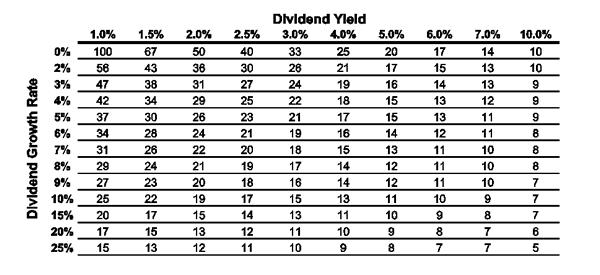# Interaction of Dividend Yield and Growth on the Payback PeriodOne cannot simply look at the price of a security and determine if the price is enticing: A \$10 stock may be more expensive than a \$100 stock when the price is related to current and expected dividend payments, growth and capital appreciation. The dividend yield is a basic, but important measure of the attractiveness of an investment. It is a simple ratio that relates the expected annual income stream to the current stock price—indicated annual dividend divided by share price. The higher the dividend yield, the greater the expected annual income. A stock trading at \$100 per share with an indicated annual dividend of \$5 per share has a dividend yield of 5% (\$5 ÷ \$100 = 0.05, or 5.0%). The yield serves as a simple benchmark for evaluating alternative investment options. It can also be used to find out how quickly you will recoup your investment by examining the dividend stream.

The payback period is a measure of how long it will take the annual dividends per share to pay back the original investment. The faster the payback, the better, assuming risk levels among your alternatives are equal. A faster payback will allow you to recover your investment more quickly and enable you to deploy the money in other assets. The longer the payback period, the greater the uncertainty of earning a positive rate of return on your investment.

Here is how it works: Assume a \$100 stock is paying a \$5 annual dividend (5% dividend yield) at the time of purchase, but no growth in the annual dividend payment is expected. It will take 20 years for your original investment to be paid back to you with dividends without regard to the future stock price or the time value of money (\$5 × 20 years = \$100). If no growth in the dividend is expected, you can simply divide the price by the annual dividend or take the reciprocal of the dividend yield (1 ÷ 0.05 = 20). A stock yielding 10% has a dividend payback period of 10 years (1 ÷ 0.10 = 10 years), while a stock with a 2% yield has a 50-year dividend payback period. The calculation is more complex if the dividend is expected to grow, but the table below makes it easy to determine the payback period for a range of dividend yields and growth rates.

The dividend payback period will be shorter if the annual dividend payment is expected to get larger over time. The dividend payback period is 26 years for a stock with a 2% current dividend yield that is expected to increase annually by 5%. Even a stock with a 1% dividend yield and a 5% dividend growth rate has a payback period of 37 years, compared to a 100-year payback period if no dividend growth is expected.

The dividend payback table illustrates the interaction between the dividend yield and dividend growth rate on the payback period. The higher the yield and the greater the growth, the quicker the payback—the ideal investment. The least desirable attributes are a low dividend yield coupled with little or no dividend growth. While it is easy for us to understand and estimate the payback period looking at just the yield, it is harder to gain an intuitive feel for the impact of dividend growth on payback period, so the table is a helpful tool. Even moderate dividend growth greatly reduces the payback period.

All things being equal, the investment with the shorter payback period is considered the better investment. It is important to remember that this table does not reinvest the dividend payments, nor does it take into account the future stock price or the time value of money. The dividend payback calculation is not impacted by stock price movement over time, it just considers the initial dividend yield and the subsequent annual growth in the dividend payment. Calculating the dividend payback period helps to determine a baseline or worst-case scenario for getting back your initial investment. Most importantly, it frames the relationship between dividend growth and dividend yield.

The Number of Years Until Dividend Payback for Initial Investment### 5 Replies to “Interaction of Dividend Yield and Growth on the Payback Period”

1.Depoling says:

Good stuff!!

2. Pingback: AAII Blog
3.Robert Anderson, Walnut Creek, CA says:

The article should also illustrate the influence of dividend reinvestment.

4.John T Headlee says:

Simplistic. What is the payback period if dividends are re-invested. E.g., T pays about 5% and in a few years the number of shars I owned incresed from 140 to 160 compounding the amount of dvidends paid. Anyone can divide one by the annual yield. Why not write an article that gives the internal rate of return that incorporates dividend re-investment?

1.Chas Mann says:

If you incorporate dividend reinvestment in your calculation, these dividend proceeds then increase invested capital, which lengthens, not shortens, your payback period (unless, of course, the dividend rate is >=100%). The correct way to calculate payback period if you are reinvesting dividends, is to ignore reinvested dividends in your equity purchases, or alternatively, calculate a separate payback period for each round of investment, to include any reinvested dividends. You are correct, however, that that calculation does not provide the investment’s IRR. The investment’s IRR, however, is a topic separate from (though related to) the payback period.